# TensorFlow从1到2（十一）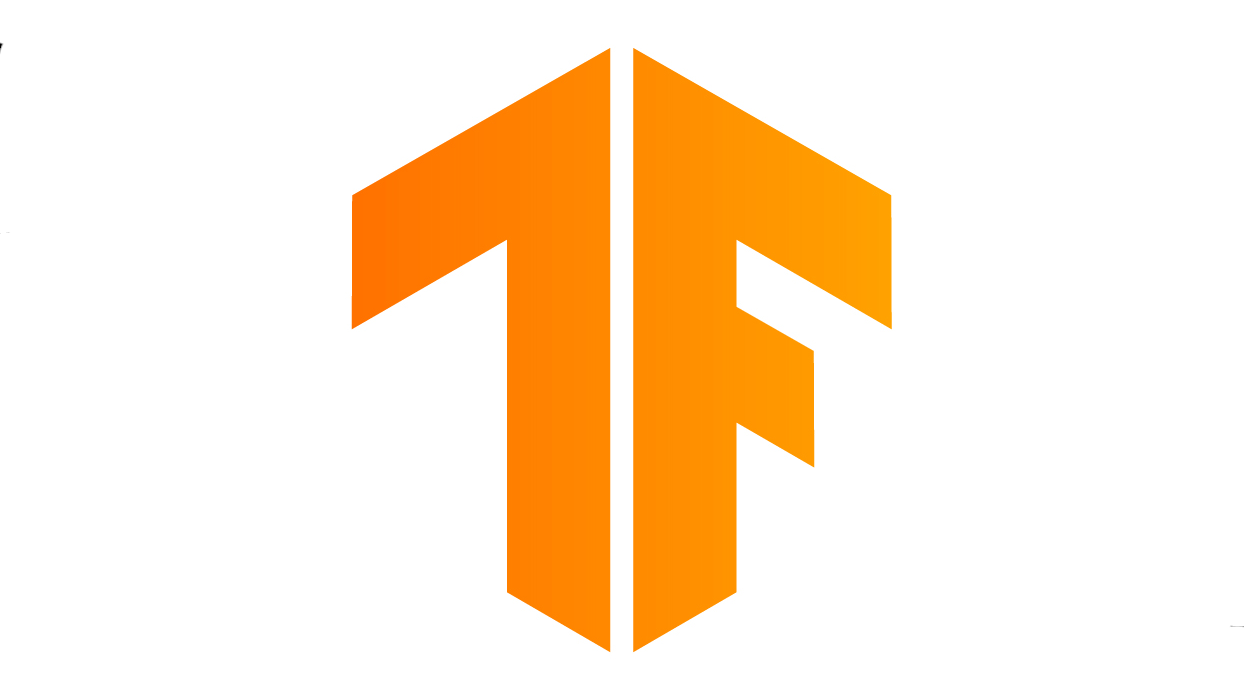#### 基本概念

“变分自动编码器”(Variational Autoencoders，缩写:VAE)的概念来自Diederik P Kingma和Max Welling的论文《Auto-Encoding Variational Bayes》。现在有了很广泛的应用，应用范围已经远远超出了当时论文的设想。不过看起来似乎，国内还没有见到什么相关产品出现。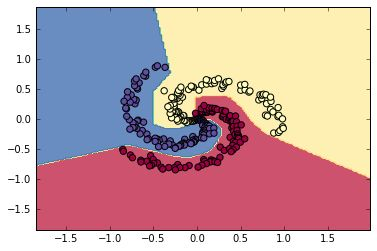#### 变分自动编码器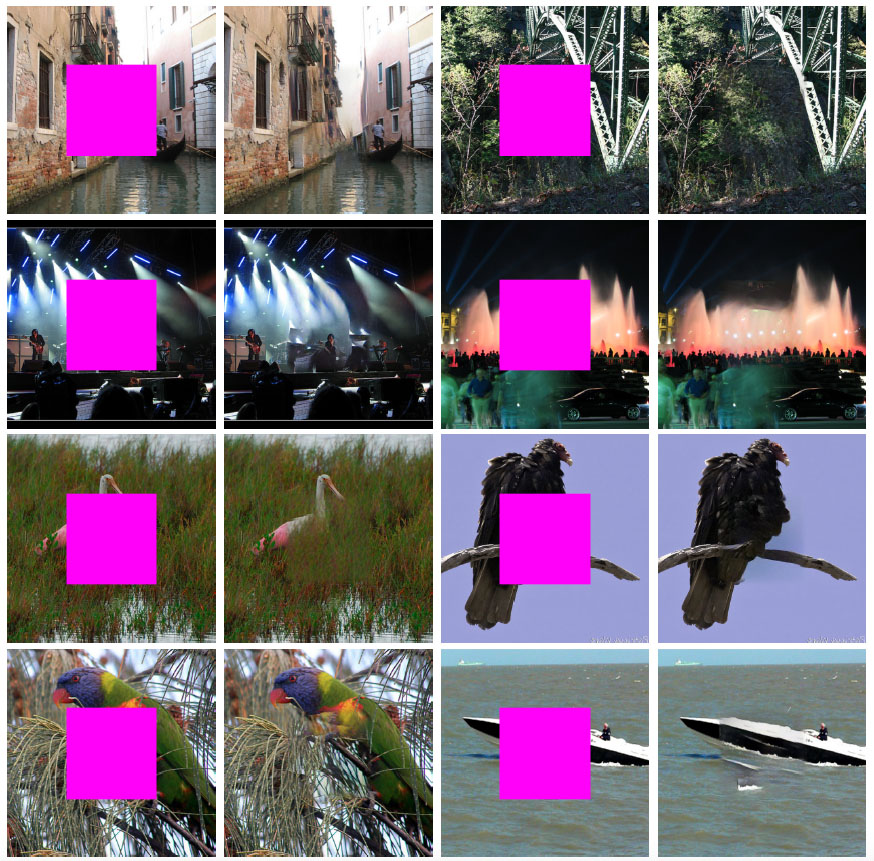#### 程序要点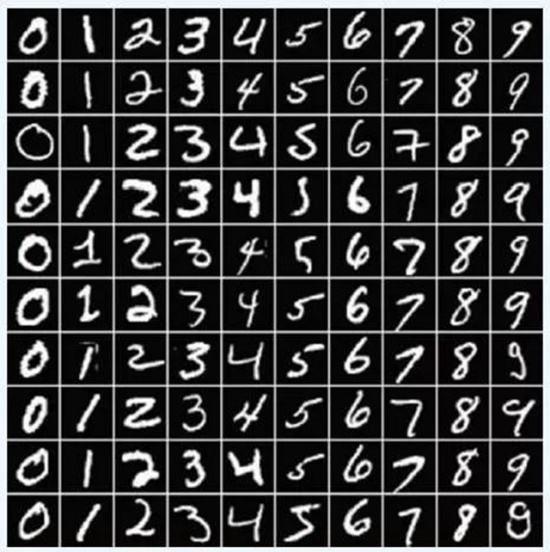(MNIST手写数字样本图片)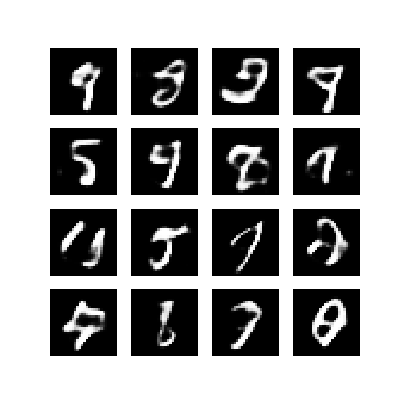(VAE经过100次训练迭代后，生成的手写数字样本图片)

``````	...
latent_dim = 50
num_examples_to_generate = 16
...
random_vector_for_generation = tf.random.normal(
shape=[num_examples_to_generate, latent_dim])
``````

``````# 计算代价值
def log_normal_pdf(sample, mean, logvar, raxis=1):
log2pi = tf.math.log(2. * np.pi)
return tf.reduce_sum(
-.5 * ((sample - mean) ** 2. * tf.exp(-logvar) + logvar + log2pi),
axis=raxis)
# 代价函数
def compute_loss(model, x):
# 编码一个批次（100）的图片
mean, logvar = model.encode(x)
# 随机生成100个均匀分布的编码向量
z = model.reparameterize(mean, logvar)
# 使用编码向量生成图片
x_logit = model.decode(z)

# 下面是代价之计算，结构很复杂，但来源是生成图片和样本图片的对比
cross_ent = tf.nn.sigmoid_cross_entropy_with_logits(logits=x_logit, labels=x)
logpx_z = -tf.reduce_sum(cross_ent, axis=[1, 2, 3])
logpz = log_normal_pdf(z, 0., 0.)
logqz_x = log_normal_pdf(z, mean, logvar)
return -tf.reduce_mean(logpx_z + logpz - logqz_x)
``````

``````    # 在向量空间内均匀分布生成100个随机编码
def reparameterize(self, mean, logvar):
eps = tf.random.normal(shape=mean.shape)
return eps * tf.exp(logvar * .5) + mean
``````

``````# 产生一幅图片，输出的时候文件名加上迭代次数
def generate_and_save_images(model, epoch, test_input):
# 生成16幅样本图片
predictions = model.sample(test_input)
# 4格*4格图片
fig = plt.figure(figsize=(4, 4))

# for i in range(predictions.shape):
# 用样本中的前16幅生成一张4x4排布的汇总图片
for i in range(4*4):
plt.subplot(4, 4, i+1)
plt.imshow(predictions[i, :, :, 0], cmap='gray')
plt.axis('off')

# 把生成的图片保存为图片文件
plt.savefig('image_at_epoch_{:04d}.png'.format(epoch))
# 也可直接显示在屏幕上，但训练过程比较慢，你不一定想等着看
# plt.show()
# 如果图片只是用于保存而非显示，则不会有用户手动“关闭”图片窗口
# plt对象也就无法关闭，所以需要显示的关闭释放内存，特别是本例中图片数量非常多
plt.close()
``````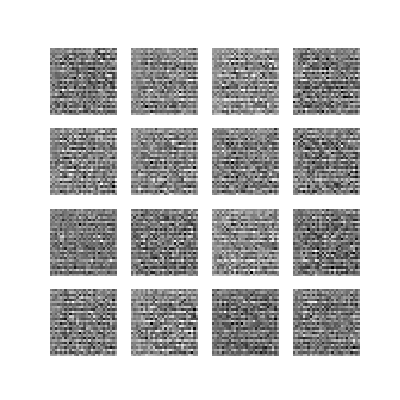``````#!/usr/bin/env python3

from __future__ import absolute_import, division, print_function, unicode_literals

# 执行前请先安装imageio库
# pip3 install imageio

import os
import time
import numpy as np
import glob
import matplotlib.pyplot as plt
import PIL
import imageio

# 遍历所有png图片，生成一张gif动图
anim_file = 'cvae-100-all.gif'
with imageio.get_writer(anim_file, mode='I') as writer:
filenames = glob.glob('image*.png')
filenames = sorted(filenames)
last = -1
for i, filename in enumerate(filenames):
frame = 2*(i**0.5)
if round(frame) > round(last):
last = frame
else:
continue
writer.append_data(image)
# 最后一张图片是最终效果图，多存一张让显示时间长一点
writer.append_data(image)
``````

#### 完整VAE代码

``````#!/usr/bin/env python3

# 引入所需库
from __future__ import absolute_import, division, print_function, unicode_literals

import tensorflow as tf

import os
import time
import numpy as np
import glob
import matplotlib.pyplot as plt

# 读取手写字体样本集
(train_images, _), (test_images, _) = tf.keras.datasets.mnist.load_data()

# 重整为：样本数x宽x高x色深 的格式
train_images = train_images.reshape(train_images.shape, 28, 28, 1).astype('float32')
test_images = test_images.reshape(test_images.shape, 28, 28, 1).astype('float32')

# 规范化数据到0-1浮点
train_images /= 255.
test_images /= 255.

# 将数据二值化，背景是0，笔画是1
train_images[train_images >= .5] = 1.
train_images[train_images < .5] = 0.
test_images[test_images >= .5] = 1.
test_images[test_images < .5] = 0.

TRAIN_BUF = 60000
BATCH_SIZE = 100

TEST_BUF = 10000

# 这里需要注意一下批次数量是100
train_dataset = tf.data.Dataset.from_tensor_slices(train_images).shuffle(TRAIN_BUF).batch(BATCH_SIZE)
test_dataset = tf.data.Dataset.from_tensor_slices(test_images).shuffle(TEST_BUF).batch(BATCH_SIZE)

class CVAE(tf.keras.Model):
def __init__(self, latent_dim):
super(CVAE, self).__init__()
self.latent_dim = latent_dim
# 推理模型，相当于Encoder，用于把手写数字图片，编码到向量
# 这里得到的不直接是向量本身，而是向量的均值和对数方差
# 原因看文中的解释
self.inference_net = tf.keras.Sequential(
[
tf.keras.layers.InputLayer(input_shape=(28, 28, 1)),
tf.keras.layers.Conv2D(
filters=32, kernel_size=3, strides=(2, 2), activation='relu'),
tf.keras.layers.Conv2D(
filters=64, kernel_size=3, strides=(2, 2), activation='relu'),
tf.keras.layers.Flatten(),
# 均值和对数方差的长度都是latent_dim，所以这里是两个
tf.keras.layers.Dense(latent_dim + latent_dim),
]
)

# 生成模型，相当于Decoder，使用编码生成对应的手写数字图片
self.generative_net = tf.keras.Sequential(
[
tf.keras.layers.InputLayer(input_shape=(latent_dim,)),
tf.keras.layers.Dense(units=7*7*32, activation=tf.nn.relu),
tf.keras.layers.Reshape(target_shape=(7, 7, 32)),
tf.keras.layers.Conv2DTranspose(
filters=64,
kernel_size=3,
strides=(2, 2),
activation='relu'),
tf.keras.layers.Conv2DTranspose(
filters=32,
kernel_size=3,
strides=(2, 2),
activation='relu'),
# No activation
tf.keras.layers.Conv2DTranspose(
]
)
# 获取一百幅样本图片
def sample(self, eps=None):
if eps is None:
eps = tf.random.normal(shape=(100, self.latent_dim))
return self.decode(eps, apply_sigmoid=True)

# 编码器
def encode(self, x):
mean, logvar = tf.split(self.inference_net(x), num_or_size_splits=2, axis=1)
# 每一步都保存一份平均值和对数方差，以便将来你可能想生成一组符合平均分布的编码
self.mean = mean
self.logvar = logvar
return mean, logvar

# 在向量空间内均匀分布生成100个随机编码
def reparameterize(self, mean, logvar):
eps = tf.random.normal(shape=mean.shape)
# tf.exp  is e^(logvar*0.5)
return eps * tf.exp(logvar * .5) + mean

# 解码器
def decode(self, z, apply_sigmoid=False):
logits = self.generative_net(z)
if apply_sigmoid:
probs = tf.sigmoid(logits)
return probs

return logits

# 代价值的计算比较复杂，是公式的编程实现
def log_normal_pdf(sample, mean, logvar, raxis=1):
log2pi = tf.math.log(2. * np.pi)
return tf.reduce_sum(
-.5 * ((sample - mean) ** 2. * tf.exp(-logvar) + logvar + log2pi),
axis=raxis)
# 代价函数
def compute_loss(model, x):
# 编码一个批次（100）的图片
mean, logvar = model.encode(x)
# 随机生成100个均匀分布的编码向量
z = model.reparameterize(mean, logvar)
# 使用编码向量生成图片
x_logit = model.decode(z)

# 下面是代价之计算，结构很复杂，但来源是生成图片和样本图片的对比
cross_ent = tf.nn.sigmoid_cross_entropy_with_logits(logits=x_logit, labels=x)
logpx_z = -tf.reduce_sum(cross_ent, axis=[1, 2, 3])
logpz = log_normal_pdf(z, 0., 0.)
logqz_x = log_normal_pdf(z, mean, logvar)
return -tf.reduce_mean(logpx_z + logpz - logqz_x)

# 进行一次训练和梯度迭代
loss = compute_loss(model, x)

# 根据梯度下降计算的结果，调整模型的权重值

# 训练迭代100次
epochs = 100
# 编码向量的维度
latent_dim = 50
# 用于生成图片的样本数，4格x4格共16幅
num_examples_to_generate = 16

# 随机生成16个编码向量，在整个程序过程中保持不变，从而可以看到
# 每次迭代，所生成的图片的效果在逐次都在优化。相同的编码会生成相同的目标数字图片
random_vector_for_generation = tf.random.normal(
shape=[num_examples_to_generate, latent_dim])
# 模型实例化
model = CVAE(latent_dim)

# 产生一幅图片，输出的时候文件名加上迭代次数
def generate_and_save_images(model, epoch, test_input):
# 生成16幅样本图片
predictions = model.sample(test_input)
# 4格*4格图片
fig = plt.figure(figsize=(4, 4))

# for i in range(predictions.shape):
# 用样本中的前16幅生成一张4x4排布的汇总图片
for i in range(4*4):
plt.subplot(4, 4, i+1)
plt.imshow(predictions[i, :, :, 0], cmap='gray')
plt.axis('off')

# 把生成的图片保存为图片文件
plt.savefig('image_at_epoch_{:04d}.png'.format(epoch))
# 也可直接显示在屏幕上，但训练过程比较慢，你不一定想等着看
# plt.show()
# 如果图片只是用于保存而非显示，则不会有用户手动“关闭”图片窗口
# plt对象也就无法关闭，所以需要显示的关闭释放内存，特别是本例中图片数量非常多
plt.close()

# 先生成第一幅、未经训练情况下的样本图片，所有的手写字符都还在随机噪点状态
generate_and_save_images(model, 0, random_vector_for_generation)

# 训练循环
for epoch in range(1, epochs + 1):
start_time = time.time()
for train_x in train_dataset:
# 训练一个批次
end_time = time.time()

# 在每个迭代循环生成一张图片和显示一次模型信息
# 可以修改为多次循环显示一次和生成一张图片
if epoch % 1 == 0:
loss = tf.keras.metrics.Mean()
for test_x in test_dataset:
loss(compute_loss(model, test_x))
elbo = -loss.result()
# 显示迭代次数、损失值、和本次迭代循环耗时
print("============================")
print(
'Epoch: {}, Test set ELBO: {}, '
'time elapse for current epoch {}'.format(
epoch,
elbo,
end_time - start_time))
# 生成一张图片保存起来
generate_and_save_images(
model, epoch, random_vector_for_generation)
``````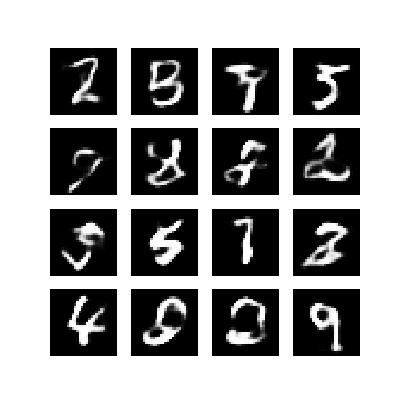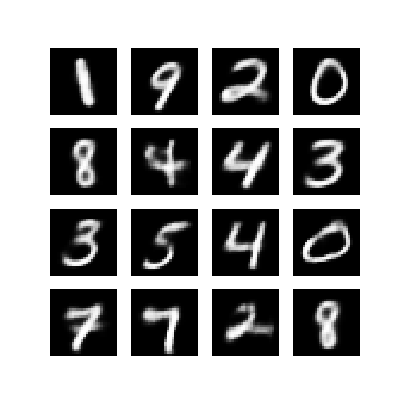（待续…）